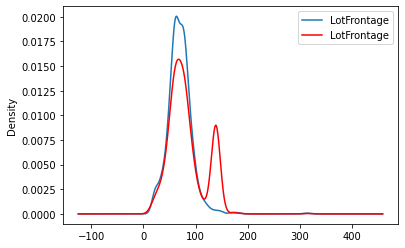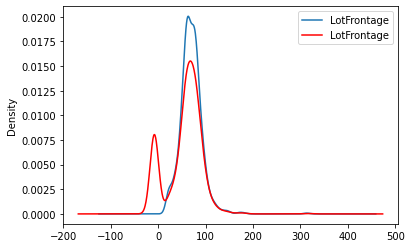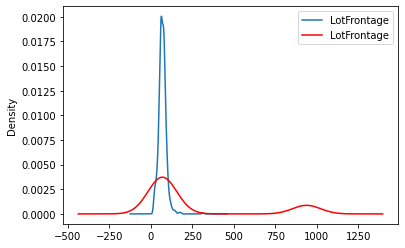# EndTailImputer¶

The EndTailImputer() replaces missing data by a value at either tail of the distribution. It automatically determines the value to be used in the imputation using the mean plus or minus a factor of the standard deviation, or using the inter-quartile range proximity rule. Alternatively, it can use a factor of the maximum value.

The EndTailImputer() is in essence, very similar to the ArbitraryNumberImputer, but it selects the value to use fr the imputation automatically, instead of having the user pre-define them.

It works only with numerical variables.

For this demonstration, we use the Ames House Prices dataset produced by Professor Dean De Cock:

Dean De Cock (2011) Ames, Iowa: Alternative to the Boston Housing Data as an End of Semester Regression Project, Journal of Statistics Education, Vol.19, No. 3

The version of the dataset used in this notebook can be obtained from Kaggle

## Version¶

In :
# Make sure you are using this
# Feature-engine version.

import feature_engine

feature_engine.__version__

Out:
'1.2.0'
In :
import numpy as np
import pandas as pd
import matplotlib.pyplot as plt

from sklearn.model_selection import train_test_split

from feature_engine.imputation import EndTailImputer


In :
# Download the data from Kaggle and store it
# in the same folder as this notebook.


Out:
Id MSSubClass MSZoning LotFrontage LotArea Street Alley LotShape LandContour Utilities ... PoolArea PoolQC Fence MiscFeature MiscVal MoSold YrSold SaleType SaleCondition SalePrice
0 1 60 RL 65.0 8450 Pave NaN Reg Lvl AllPub ... 0 NaN NaN NaN 0 2 2008 WD Normal 208500
1 2 20 RL 80.0 9600 Pave NaN Reg Lvl AllPub ... 0 NaN NaN NaN 0 5 2007 WD Normal 181500
2 3 60 RL 68.0 11250 Pave NaN IR1 Lvl AllPub ... 0 NaN NaN NaN 0 9 2008 WD Normal 223500
3 4 70 RL 60.0 9550 Pave NaN IR1 Lvl AllPub ... 0 NaN NaN NaN 0 2 2006 WD Abnorml 140000
4 5 60 RL 84.0 14260 Pave NaN IR1 Lvl AllPub ... 0 NaN NaN NaN 0 12 2008 WD Normal 250000

5 rows × 81 columns

In :
# Separate the data into train and test sets.

X_train, X_test, y_train, y_test = train_test_split(
data.drop(['Id', 'SalePrice'], axis=1),
data['SalePrice'],
test_size=0.3,
random_state=0,
)

X_train.shape, X_test.shape

Out:
((1022, 79), (438, 79))

## Check missing data¶

In :
# numerical variables with missing data

X_train[['LotFrontage', 'MasVnrArea']].isnull().mean()

Out:
LotFrontage    0.184932
MasVnrArea     0.004892
dtype: float64

The EndTailImputer can replace NA with a value at the left or right end of the distribution.

In addition, it uses 3 different methods to identify the imputation values.

In the following cells, we show how to use each method.

## Gaussian, right tail¶

Let's begin by finding the values automatically at the right tail, by using the mean and the standard deviation.

In :
imputer = EndTailImputer(

# uses mean and standard deviation to determine the value
imputation_method='gaussian',

# value at right tail of distribution
tail='right',

# multiply the std by 3
fold=3,

# the variables to impute
variables=['LotFrontage', 'MasVnrArea'],
)

In :
# find the imputation values

imputer.fit(X_train)

Out:
EndTailImputer(variables=['LotFrontage', 'MasVnrArea'])
In :
# The values for the imputation

imputer.imputer_dict_

Out:
{'LotFrontage': 138.9022201686726, 'MasVnrArea': 648.3947111415165}

Note that we use different values for different variables.

In :
# impute the data

train_t = imputer.transform(X_train)
test_t = imputer.transform(X_test)

In :
# check we no longer have NA

train_t['LotFrontage'].isnull().sum()

Out:
0
In :
# The variable distribution changed slightly with
# more values accumulating towards the right tail

fig = plt.figure()
X_train['LotFrontage'].plot(kind='kde', ax=ax)
train_t['LotFrontage'].plot(kind='kde', ax=ax, color='red')
lines, labels = ax.get_legend_handles_labels()
ax.legend(lines, labels, loc='best')

Out:
<matplotlib.legend.Legend at 0xef4b0e6790>## IQR, left tail¶

Now, we will impute variables with values at the left tail. The values are identified using the inter-quartile range proximity rule.

The IQR rule is better suited for skewed variables.

In :
imputer = EndTailImputer(

# uses the inter-quartile range proximity rule
imputation_method='iqr',

# determines values at the left tail of the distribution
tail='left',

# multiplies the IQR by 3
fold=3,

# the variables to impute
variables=['LotFrontage', 'MasVnrArea'],
)

In :
# finds the imputation values

imputer.fit(X_train)

Out:
EndTailImputer(imputation_method='iqr', tail='left',
variables=['LotFrontage', 'MasVnrArea'])
In :
# imputation values per variable

imputer.imputer_dict_

Out:
{'LotFrontage': -8.0, 'MasVnrArea': -510.0}
In :
# transform the data

train_t = imputer.transform(X_train)
test_t = imputer.transform(X_test)

In :
# Check we have no NA after the transformation

train_t[['LotFrontage', 'MasVnrArea']].isnull().sum()

Out:
LotFrontage    0
MasVnrArea     0
dtype: int64
In :
# The variable distribution changed with the
# transformation, with more values
# accumulating towards the left tail.

fig = plt.figure()
X_train['LotFrontage'].plot(kind='kde', ax=ax)
train_t['LotFrontage'].plot(kind='kde', ax=ax, color='red')
lines, labels = ax.get_legend_handles_labels()
ax.legend(lines, labels, loc='best')

Out:
<matplotlib.legend.Legend at 0xef4d27e9a0>## Impute with the maximum value¶

We can find imputation values with a factor of the maximum variable value.

In :
imputer = EndTailImputer(

# imputes beyond the maximum value
imputation_method='max',

# multiplies the maximum value by 3
fold=3,

# the variables to impute
variables=['LotFrontage', 'MasVnrArea'],
)

In :
# find imputation values

imputer.fit(X_train)

Out:
EndTailImputer(imputation_method='max', variables=['LotFrontage', 'MasVnrArea'])
In :
# The imputation values.

imputer.imputer_dict_

Out:
{'LotFrontage': 939.0, 'MasVnrArea': 4800.0}
In :
# the maximum values of the variables,
# note how the imputer multiplied them by 3
# to determine the imputation values.

X_train[imputer.variables_].max()

Out:
LotFrontage     313.0
MasVnrArea     1600.0
dtype: float64
In :
# impute the data

train_t = imputer.transform(X_train)
test_t = imputer.transform(X_test)

In :
# Check we have no NA  in the imputed data

train_t[['LotFrontage', 'MasVnrArea']].isnull().sum()

Out:
LotFrontage    0
MasVnrArea     0
dtype: int64
In :
# The variable distribution changed with the
# transformation, with now more values
# beyond the maximum.

fig = plt.figure()
X_train['LotFrontage'].plot(kind='kde', ax=ax)
train_t['LotFrontage'].plot(kind='kde', ax=ax, color='red')
lines, labels = ax.get_legend_handles_labels()
ax.legend(lines, labels, loc='best')

Out:
<matplotlib.legend.Legend at 0xef4d2fd520>## Automatically impute all variables¶

As with all Feature-engine transformers, the EndTailImputer can also find and impute all numerical variables in the data.

In :
# Start the imputer

imputer = EndTailImputer()

In :
# Check the default parameters

# how to find the imputation value
imputer.imputation_method

Out:
'gaussian'
In :
# which tail to use

imputer.tail

Out:
'right'
In :
# how far out
imputer.fold

Out:
3
In :
# Find variables and imputation values

imputer.fit(X_train)

Out:
EndTailImputer()
In :
# The variables to impute

imputer.variables_

Out:
['MSSubClass',
'LotFrontage',
'LotArea',
'OverallQual',
'OverallCond',
'YearBuilt',
'MasVnrArea',
'BsmtFinSF1',
'BsmtFinSF2',
'BsmtUnfSF',
'TotalBsmtSF',
'1stFlrSF',
'2ndFlrSF',
'LowQualFinSF',
'GrLivArea',
'BsmtFullBath',
'BsmtHalfBath',
'FullBath',
'HalfBath',
'BedroomAbvGr',
'KitchenAbvGr',
'TotRmsAbvGrd',
'Fireplaces',
'GarageYrBlt',
'GarageCars',
'GarageArea',
'WoodDeckSF',
'OpenPorchSF',
'EnclosedPorch',
'3SsnPorch',
'ScreenPorch',
'PoolArea',
'MiscVal',
'MoSold',
'YrSold']
In :
#  The imputation values

imputer.imputer_dict_

Out:
{'MSSubClass': 183.0960051903714,
'LotFrontage': 138.9022201686726,
'LotArea': 41441.796589850215,
'OverallQual': 10.152919665538322,
'OverallCond': 8.918356149675976,
'YearBuilt': 2061.66731604675,
'MasVnrArea': 648.3947111415165,
'BsmtFinSF1': 1732.0016007094835,
'BsmtFinSF2': 520.9882766560984,
'BsmtUnfSF': 1865.113698435333,
'TotalBsmtSF': 2286.0497168767233,
'1stFlrSF': 2283.6805173062803,
'2ndFlrSF': 1677.2392305771546,
'LowQualFinSF': 149.0787736885176,
'GrLivArea': 3075.569310556133,
'BsmtFullBath': 1.9636856192070633,
'BsmtHalfBath': 0.7637721815299992,
'FullBath': 3.2012740993879882,
'HalfBath': 1.877166869732324,
'BedroomAbvGr': 5.303758597292265,
'KitchenAbvGr': 1.7084277213255645,
'TotRmsAbvGrd': 11.395793721778118,
'Fireplaces': 2.519529226227064,
'GarageYrBlt': 2052.9707419772235,
'GarageCars': 3.966386813249906,
'GarageArea': 1095.8302008827814,
'WoodDeckSF': 480.04361090824267,
'OpenPorchSF': 250.26561495660084,
'EnclosedPorch': 216.43485488519244,
'3SsnPorch': 89.5229867716376,
'ScreenPorch': 184.35773738383577,
'PoolArea': 101.82445982535369,
'MiscVal': 1817.7712851835915,
'MoSold': 14.42955308807171,
'YrSold': 2011.8643245428148}
In :
# impute the data

train_t = imputer.transform(X_train)
test_t = imputer.transform(X_test)

In :
# Sanity check:

# No numerical variable with NA is  left in the
# transformed data.

[v for v in train_t.columns if train_t[v].dtypes !=
'O' and train_t[v].isnull().sum() > 1]

Out:
[]
In [ ]: